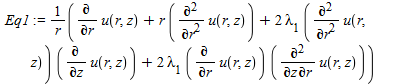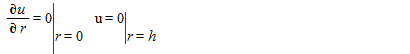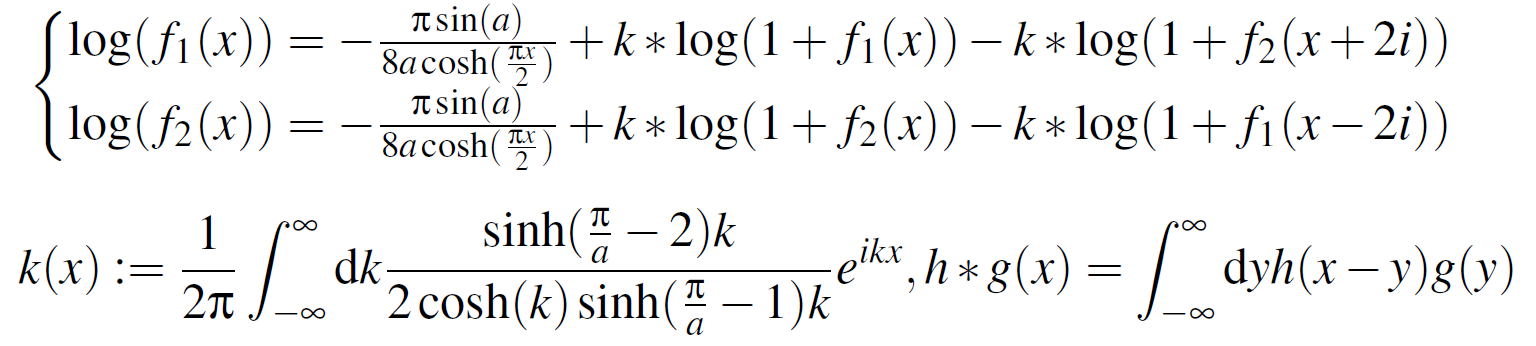## How to obtain a solution using pdsolve...

Dear maple users

Greetings.

In this code, I am solving the PDEs via perturbation method.

There is some mistake in the boundary condition and pdsolve.

Kindly help me that to get the solution for this PDE via perturbation method.BC:Code: JVB.mw

## Can someone solve this questions on maple...## Can someone solve all of this questions into maple...eq1 := diff(h(t), [$(t, nu)])+(1/4)*h(t) = 8/3*(1/(sqrt(Pi)*t^(1/2))-(3/32)*t^2+(3/16)*exp(-t)-1); with ics h^k (0)=0 for k=0..n ## how to plot this graphs... Asked by: how are please I want to help In some graphs in maple the graph in the picture thank you) ## convolution , analytic vs numeric... Asked by: Download analy_numer.mw Hello, I am trying to compute the convolution integral numerically and compare the result with the analytic result. My numerics is giving terrible results: ## How to i solve the question B given in maple... Asked by:## How do i solve this ordinary differential equation... Asked by:## Hello, i want to ask can we convert matlab code to... Asked by: clc;clear; % Define i1(t) and i2(t) as symbolic variable syms i1(t) i2(t) % Given differential equation ode1 = diff(i1) == 0.5*i1 + -3*i2 +5*exp(-2*t); ode2 = diff(i2) == 2*i1 - 6*i2; odes = [ode1; ode2]; % Define initial conditions cond1 = i1(0) == 1; cond2 = i2(0) == -1; conds = [cond1; cond2]; % Solution of system of differential equation [i1(t), i2(t)] = dsolve(odes,conds) fplot(i1(t)) hold on fplot(i2(t)) legend('i1(t)','i2(t)') Output: i1(t) = exp((t*(73^(1/2) - 11))/4)*(73^(1/2)/8 + 13/8)*((57*73^(1/2))/292 - exp((3*t)/4 - (73^(1/2)*t)/4)*((15*73^(1/2))/292 + 5/4) + 3/4) - exp(-(t*(73^(1/2) + 11))/4)*(73^(1/2)/8 - 13/8)*(exp((3*t)/4 + (73^(1/2)*t)/4)*((15*73^(1/2))/292 - 5/4) + (3*73^(1/2)*(73^(1/2) - 19))/292) i2(t) = exp(-(t*(73^(1/2) + 11))/4)*(exp((3*t)/4 + (73^(1/2)*t)/4)*((15*73^(1/2))/292 - 5/4) + (3*73^(1/2)*(73^(1/2) - 19))/292) + exp((t*(73^(1/2) - 11))/4)*((57*73^(1/2))/292 - exp((3*t)/4 - (73^(1/2)*t)/4)*((15*73^(1/2))/292 + 5/4) + 3/4) ## Errors in PDE model plot... Asked by: ## How do I solve numerically a set of non-linear int... Asked by: Hey everyone, f_1 and f_2 are satisfying the set of non-linear integral equations I have attached to this message. I know that I need to solve them numerically by iterations. Probably, the first guest of the function f_1 and f_2 is the driving term. a is just a parameter which can be fixed (I guess smaller than \pi/4). * is the convolution product and k is the momentum space parameter. I learnt that in order to solve them I should solve them in the Fourier space. I know also that I need to discretize these function in the “real ” space between {-L,+L} before applying the FFT or one of its relatives. Thank you for any suggestions or leads.## Statistical model not giving a proper fit to a dat... Asked by: ## dsolve not working for N=1 or N=2.... Asked by: Dear maple users. Greetings for the day. I hope you are all fine and safe. In the below mention code, I need to plot "ax" at 0..1 when N=1 and N=2. But the code only working for the N=0 case. How to tackle this situation and plot the function for various values of "ax" at ax=0..1. waiting for your reply. JBV.mw Code: restart; PDEtools[declare](f(x), t(x), g(x), prime = (x)); N := 2; m := .2; pa := 3.14*(1/3); ax := ax; h2 := 1+.2*ax+.3*sin((2*3.14)*(ax-.2)); h1 := -1-.2*ax-.1*sin((2*3.14)*(ax-.2)+pa); a2 := 1.4+.1*sin((2*3.14)*(ax-.2))+.3*sin((2*3.14)*(ax-.2)+pa); f(x):=sum(p^j*f[j](x),j=0..N); t(x):=sum(p^j*t[j](x),j=0..N); g(x):=sum(p^j*g[j](x),j=0..N); Eq1 := (1-p)*(diff(f(x), $(x, 4)))+p*((1+.2)*(diff(f(x), $(x, 4)))-(.2*(1/3))*(diff((diff(f(x), $(x, 2)))^3, $(x, 2)))-2*(diff(f(x), $(x, 2)))+diff(t(x), $(x, 1))+diff(g(x), $(x, 1)));

Eq2 := (1-p)*(1+1.2)*(diff(t(x), $(x, 2)))+p*((1+1.2)*(diff(t(x), $(x, 2)))+.1*(diff(t(x), $(x, 1)))*(diff(g(x), $(x, 1)))+.2*(diff(t(x), $(x, 1)))^2+.5*(diff(f(x), $(x, 1)))^2);

Eq3 := (1-p)*(diff(g(x), $(x, 2)))+p*(diff(g(x), $(x, 2))+diff(t(x), $(x, 2))); for j from 0 to N do equ1[j] := coeff(Eq1, p, j) = 0; equ2[j] := coeff(Eq2, p, j) = 0; equ3[j] := coeff(Eq3, p, j) = 0; end do; con := f(h2) = (1/2)*a2, (D(f))(h2) = 0, f(h1) = -(1/2)*a2, (D(f))(h1) = 0; con := t(h2) = 1, t(h1) = 0; con := g(h2) = 1, g(h1) = 0; for i to N do con[i] := f[i](h2) = 0, (D(f[i]))(h2) = 0, f[i](h1) = 0, (D(f[i]))(h1) = 0; con[i] := t[i](h2) = 0, t[i](h1) = 0; con[i] := g[i](h2) = 0, g[i](h1) = 0 end do; for i from 0 to N do P:=dsolve({equ1[i],equ2[i],equ3[i],con[i],con[i],con[i]},{f[i](x),t[i](x),g[i](x)}): f[i](x):=rhs(P); t[i](x):=rhs(P); g[i](x):=rhs(P); end do: f(x):=evalf(simplify(sum(f[n](x),n=0..N))); Am := (1+.2)*(diff(f(x), $(x, 3)));
with(plots);

display(plot(eval(Am, x = .6), ax = 0 .. 1, numpoints = 200, color = blue));

## How do i clear this error...

blessing.mw please i really need your help on this, i have been trying to fix this error for a while now.

## How to find triplets in Lie algebra at which Jacob...

Dear all

I have  Lie commutations for vectors e1, e2, e3, e4, e5, e6 as follow:

[e1, e3] = e3, [e1, e4] = e4, [e1, e5] = e5, [e1, e6] = e6, [e2, e3] = -e5, [e2, e4] = e6, [e3, e5] = e6

for which the command

Query("Jacobi")

returns the false result, which means, the vectors are not closed under Jacobi's identity. How can I find vector triplets for which Jacobi's identity does not hold?